## Example Questions

### Example Question #164 : Geometry

The perimeter of a rectangle is 14, and the diagonal connecting two vertices is 5.

Quantity A: 13

Quantity B: The area of the rectangle

Quantity A is greater.

Quantity B is greater.

The two quantities are equal.

The relationship between A and B cannot be determined.

Quantity A is greater.

Explanation:

One potentially helpful first step is to draw the rectangle described in the problem statement: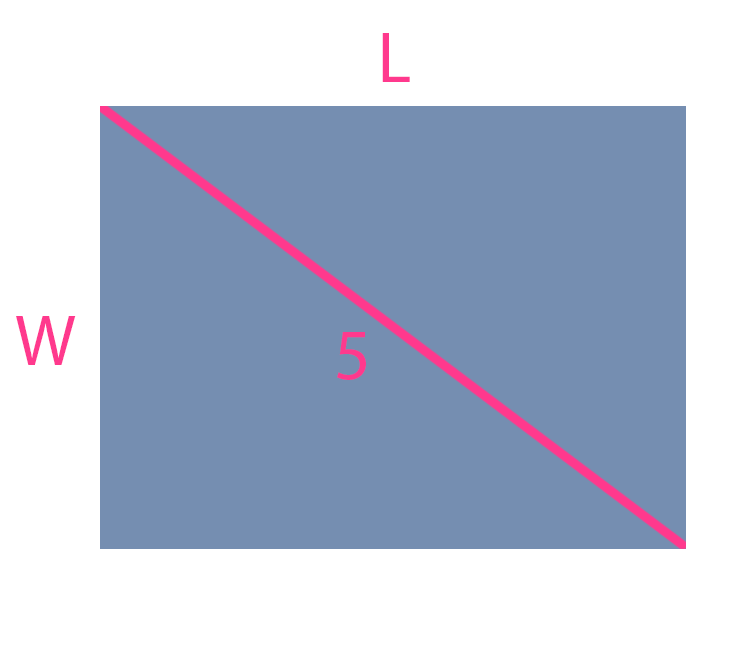After that, it's a matter of using the other information given. The perimeter is given as 14, and can be written in terms of the length and width of the rectangle: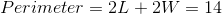Furthermore, notice that the diagonal forms the hypotenuse of a right triangle. The Pythagorean Theorem may be applied: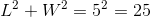This provides two equations and two unknowns. Redefining the first equation to isolategives: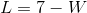Plugging this into the second equation in turn gives: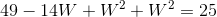Which can be reduced to: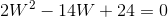or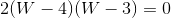Note that there are two possibile values for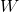; 3 or 4. The one chosen is irrelevant. Choosing a value 3, it is possible to then find a value for: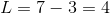This in turn allows for the definition of the rectangle's area: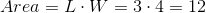So Quantity B is 12, which is less than Quantity A.

### Example Question #165 : Geometry

One rectangle has sides of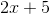and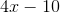. Which of the following pairs could be the sides of a rectangle similar to this one?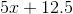and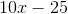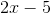and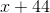and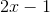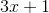and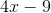andExplanation:

For this problem, you need to find the pair of sides that would reduce to the same ratio as the original set of sides. This is a little tricky at first, but consider the set:andFor this, you have: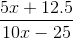Now, if you factor out, you have: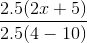Thus, the proportions are the same, meaning that the two rectangles would be similar.

### Example Question #1361 : Gre Quantitative Reasoning

One rectangle has a height ofand a width of. Which of the following is a possible perimeter of a similar rectangle, having one side that is?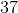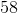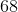Explanation:

Based on the information given, we know thatcould be either the longer or the shorter side of the similar rectangle. Similar rectangles have proportional sides. We might need to test both, but let us begin with the easier proportion, namely: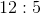as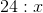For this proportion, you really do not even need fractions. You know thatmust be.

This means that the figure would have a perimeter of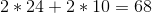Luckily, this is one of the answers!

Tired of practice problems?

Try live online GRE prep today.